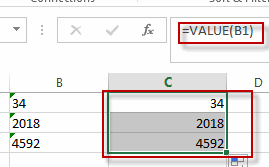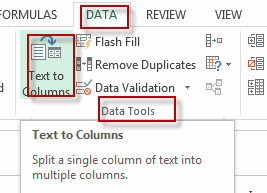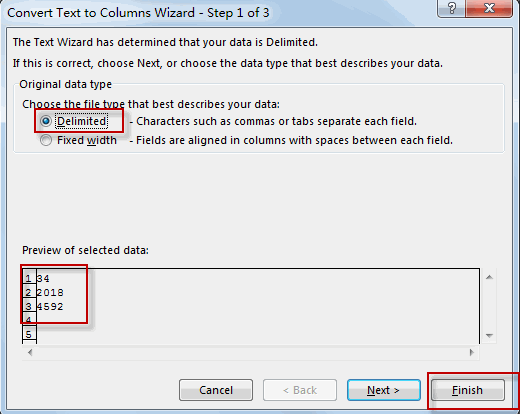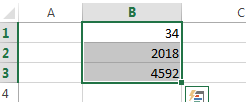# Remove Apostrophe or Text Indicator

This post will guide you how to remove the apostrophe (‘) from the text values in your worksheet in excel. How do I remove text indicator from a text string in a cell in excel. How to use an excel formula to remove text indicator quickly. How to remove leading Apostrophe from a text value in a cell.

## Remove Apostrophe with Excel Formula

If you want to remove the text indicator (‘) or the leading Apostrophe from a text string in one cell, you can create a formula based on the VALUE function.Just like this:

`=VALUE(B1)`

You just need to type this formula into the formula box of the cell C1, then press Enter key in your keyboard. And drag the AutoFill Handler over other cells to apply this formula.

Let’s see the result:## Remove Apostrophe with Text to Columns Function

You can also use the Text to Columns function to remove the text indicator from text values in one cell, just do the following steps:

#1 select the cells that you want to remove the Apostrophe or text indicator, such as: B1:B3

#2 go to DATA tab, click Text to Columns command under Data Tools group. And the Convert Text to Columns Wizard dialog will appear.#3 select the Delimited radio button, and then click Finish button.#4 you will see the text indicators have been removed.### Related Functions

• Excel VALUE function
The Excel VALUE function converts a text value that represents a number to a numeric value.The syntax of the VALUE function is as below:= VALUE  (text)…
Related Posts

If Cell Contains Either Text1 or Text2

IF function is frequently used in Excel worksheet to return “true value” or “false value” based on the logical test result. If you want to see if cell contains certain substring1 like “abc” or substring2 like “def”, and returns true ...

If Cell Contains Certain Text OR Equals Certain Text

IF cell equals certain text IF function is frequently used in Excel worksheet to return “true value” or “false value” based on the logical test result. If you want to test values to see if they equal certain text like ...

VLOOKUP From Another Sheet Not Working

In the previous post, you should know that how to fix or remove the #N/A error when using VLOOKUP formula to lookup value from another sheet. And this post will show you reasons why your VLOOKUP formula is not working ...

If Cell Begins with One of Three Supplied Characters

If you want to test values to see if they begin with some given specific characters like “x”, ”y”, or “z”, you can create a formula with COUNTIF and SUM functions to return results. EXAMPLE You can see “TRUE” or ...

Fix #N/A Error For VLOOKUP From Another Sheet

This post will show you how to fix the #N/A error why it occurs when you extract values from another sheet using VLOOKUP function in Excel 2016,2013,2010 or other Excel versions. How can you correct a #N/A error in VLOOKUP ...

How to Average Only Positive or Negative Numbers of a Range

Suppose both positive numbers and negative numbers exist in a table. If we want to know the average of only positive numbers in this table, we can create a formula to get average of all positive numbers with all negative ...

Sort Positive Numbers and Negative Numbers by Absolute Values

If both positive numbers and negative numbers exist in the same column, when sorting them by absolute values, we can sort them with the help of ABS function and helper column. In this article, we will show you the way ...

Get Employee Information by VLOOKUP

VLOOKUP is one of the key functions among all lookup & reference functions in Excel. Today, in this article, we will show you the way to apply VLOOKUP to retrieve employee information. I hope this article will help you in ...

VLOOKUP with Two Lookup Tables

VLOOKUP is one of the key functions among all lookup & reference functions in Excel. Today we will show you the application of VLOOKUP function when there are two lookup tables. EXAMPLE Table1 and table2 record the rates of Y2020 ...

VLOOKUP with Multiple Lookup Values

VLOOKUP is one of the key functions among all lookup & reference functions in Excel. It can scan and retrieve data from a static or dynamic table based on your lookup value. It can perform approximate match or exact match ...

Sidebar